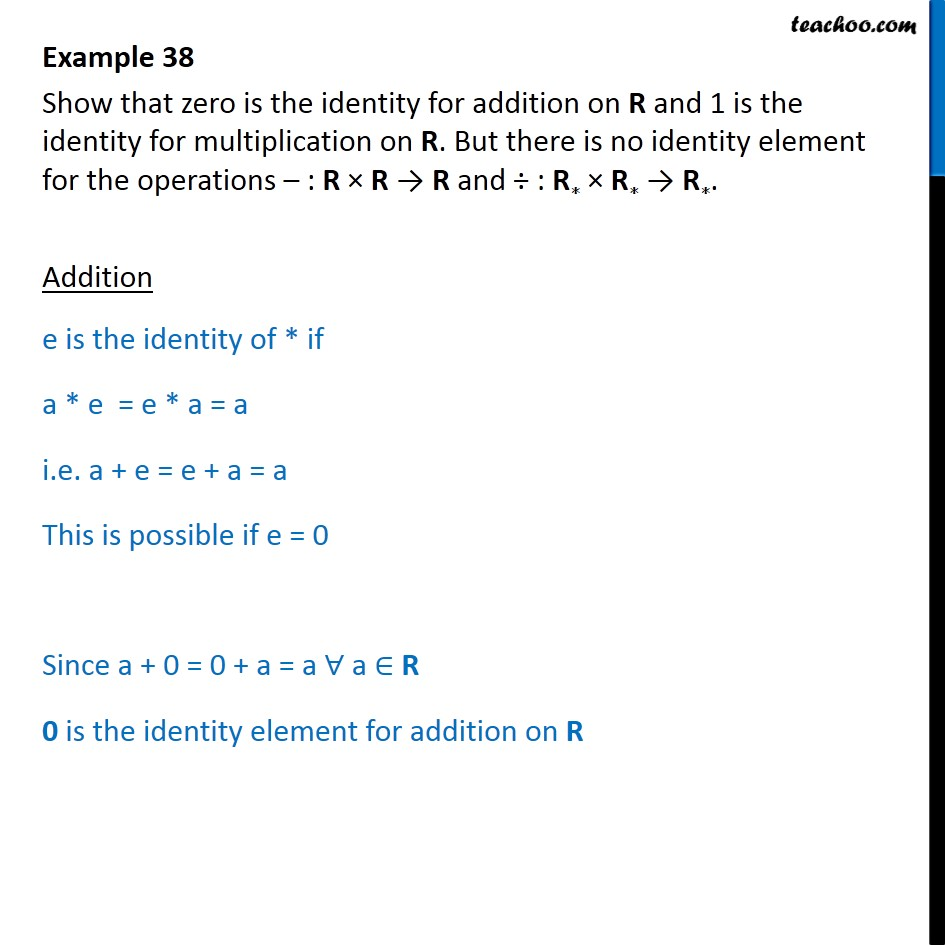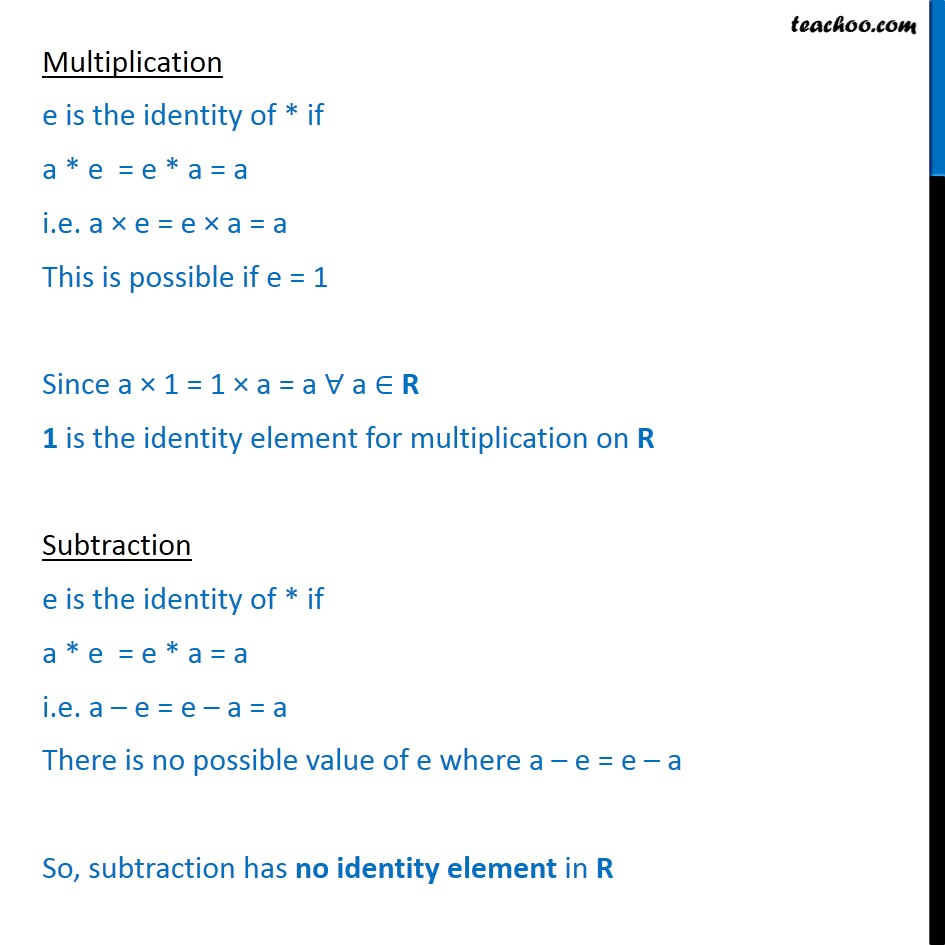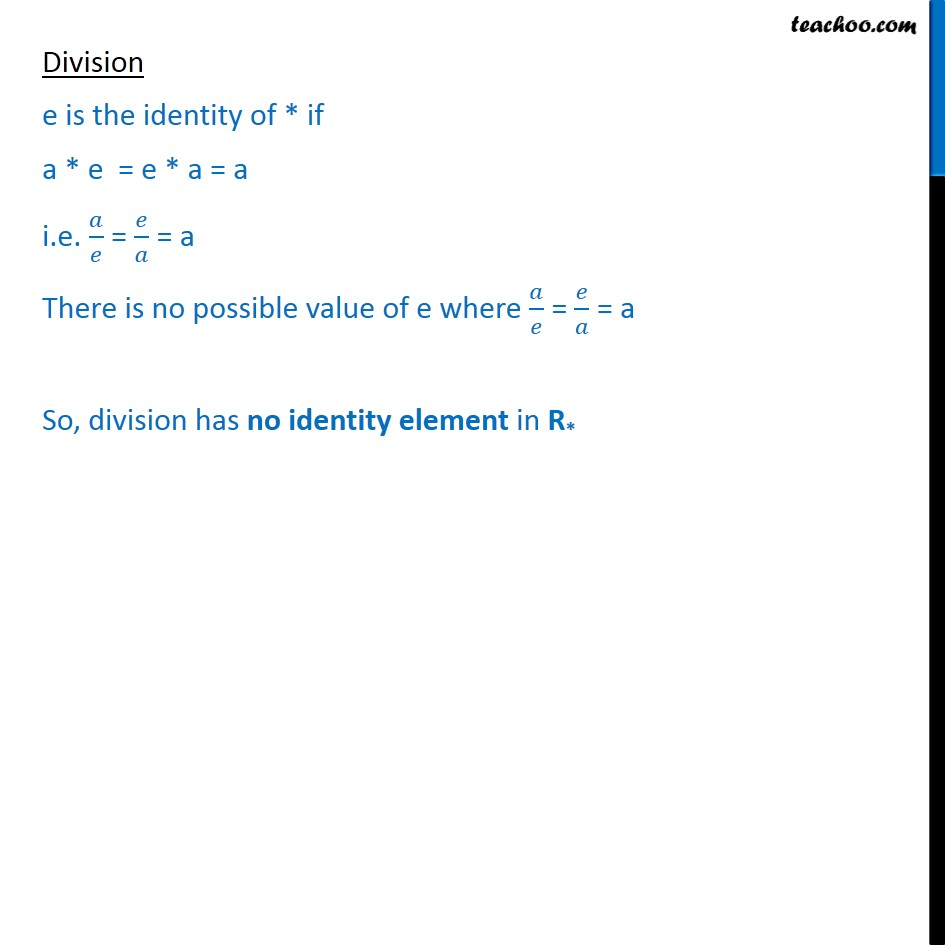Examples

Chapter 1 Class 12 Relation and Functions
Serial order wiseMaths Crash Course - Live lectures + all videos + Real time Doubt solving!

### Transcript

Example 38 Show that zero is the identity for addition on R and 1 is the identity for multiplication on R. But there is no identity element for the operations : R R R and : R R R . Addition e is the identity of * if a * e = e * a = a i.e. a + e = e + a = a This is possible if e = 0 Since a + 0 = 0 + a = a a R 0 is the identity element for addition on R Multiplication e is the identity of * if a * e = e * a = a i.e. a e = e a = a This is possible if e = 1 Since a 1 = 1 a = a a R 1 is the identity element for multiplication on R Subtraction e is the identity of * if a * e = e * a = a i.e. a e = e a = a There is no possible value of e where a e = e a So, subtraction has no identity element in R Division e is the identity of * if a * e = e * a = a i.e. = = a There is no possible value of e where = = a So, division has no identity element in R*